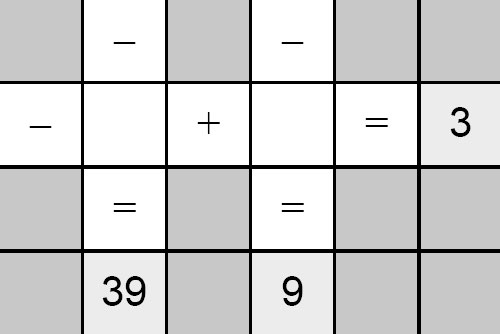# Printable Mathdoku### Printable Mathdoku

Mathdoku puzzles are kind of a mix between a math puzzle and a Sudoku puzzle. You must enter the numbers 1 through 9 into each empty white square, using each number exactly once, so that all six mathematical equations on the grid are fulfilled.

Each puzzle has one and only one unique solution, and most can be solved using only pure logical deduction – guessing and trial-and-error methods are only occasionally required for the most difficult of the “Diabolical” puzzles.

Mathdoku Puzzles